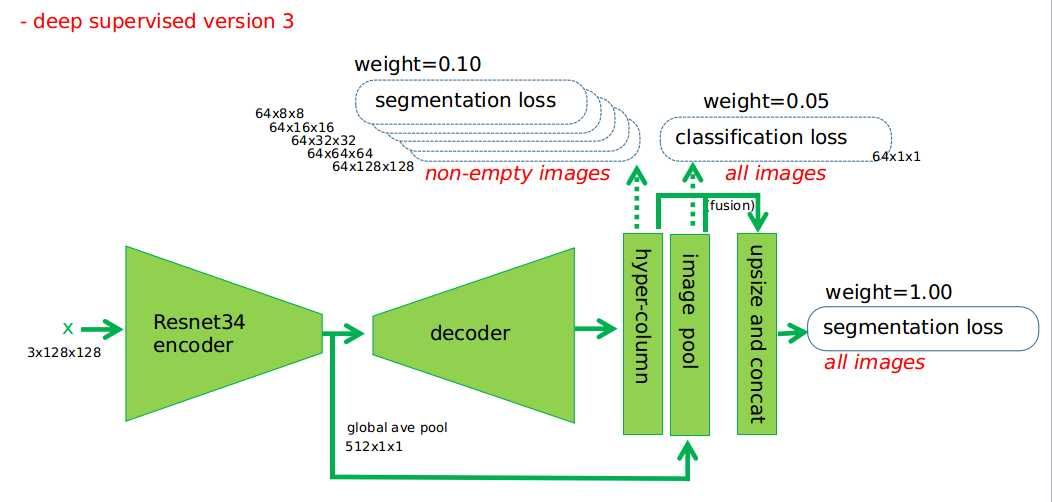# Computer Vision-Semantic segmentation (II.)

Source: Internet
Author: User

Introduction

A lot of u-net-like neural networks have been put forward.

U-net is suitable for medical image segmentation and natural image generation.

In Medical image segmentation performance is good:

1. The lack of information to improve on-the-sample is due to the use of the underlying features (same resolution Cascade).

2. Medical image data is generally less, the underlying features are actually very important.

Not only medical image, for two classification of semantic segmentation problem, class unet structure have achieved good results. Linknet, large kernel and tiramisu models are also good, but not as class unet structures

First, loss function

1. The most common loss function is binary cross entropy loss combined dice Coeff loss
The former is a loss function at the pixel level
The latter is the loss function of the image level or batch level, and is suitable for the problem based on IOU as the evaluation index.

2. Online bootstrapped cross entropy loss
such as FRNN, a difficult sample of mining

3. Lovasz loss
From the paper the Lovasz-softmax loss:a tractable surrogate for the optimization of the intersection-over-union measure in neural Networks
It is also suitable to use IOU as the evaluation index problem.

Second, the Backbone of the network

More popular Backbone such as se-resnext101,se-resnext50,se-resnet101, I think in the data set is not particularly sufficient circumstances, the difference is not small.

Due to the limitation of memory, I use ResNet34
Before doing some example detection, instance segmentation problem, with ResNet50 effect is similar to ResNet101.

Third, based on the Attention unet

The SE structure of

Concurrent Spatial and Channel Squeeze & excitation in Fully convolutional Networks
Se-net is for feature m The different channel in APS is weighted processing.
This attention is generalized in this paper, using Cselayer in Se-net, Sselayer weighted by different position, and scselayer in combination of two weights
The experiments in the paper show that these attention-gated structure, placed in different stages of encoder and decoder, than without Attention, the effect is better

```
class cSELayer(nn.Module):
def __init__(self, channel, reduction=2):
super(cSELayer, self).__init__()
self.fc = nn.Sequential(
nn.Linear(channel, channel // reduction),
nn.ELU(inplace=True),
nn.Linear(channel // reduction, channel),
nn.Sigmoid()
)

def forward(self, x):
b, c, _, _ = x.size()
y = self.avg_pool(x).view(b, c)
y = self.fc(y).view(b, c, 1, 1)
return x * y

class sSELayer(nn.Module):
def __init__(self, channel):
super(sSELayer, self).__init__()
self.fc = nn.Conv2d(channel, 1, kernel_size=1)
self.sigmoid = nn.Sigmoid()

def forward(self, x):
y = self.fc(x)
y = self.sigmoid(y)
return x * y

class scSELayer(nn.Module):
def __init__(self, channels, reduction=2):
super(scSELayer, self).__init__()
self.sSE = sSELayer(channels)
self.cSE = cSELayer(channels, reduction=reduction)

def forward(self, x):
sx = self.sSE(x)
cx = self.cSE(x)
x = sx + cx
return x
```

```

class Dblock(nn.Module):
def __init__(self, channel):
super(Dblock, self).__init__()
self.dilate1 = nn.Conv2d(channel, channel, kernel_size=3, dilation=1, padding=1)
self.dilate2 = nn.Conv2d(channel, channel, kernel_size=3, dilation=2, padding=2)
self.dilate3 = nn.Conv2d(channel, channel, kernel_size=3, dilation=4, padding=4)
for m in self.modules():
if isinstance(m, nn.Conv2d):
if m.bias is not None:
m.bias.data.zero_()

def forward(self, x):
dilate1_out = F.relu(self.dilate1(x), inplace=True)
dilate2_out = F.relu(self.dilate2(dilate1_out), inplace=True)
dilate3_out = F.relu(self.dilate3(dilate2_out), inplace=True)

out = x + dilate1_out + dilate2_out + dilate3_out
return out

```

Ocnet:object Context Network for Scene parsing
For semantic segmentation, the model needs both the contextual information of high latitude (global information) and the resolution capability (that is, the local information of the picture). Unet through concatenate to improve the image of the local information. So how do you get better global information? The center block in the middle of the unet structure is discussed in Ocnet paper.

Fifth, Hyper columns

Hypercolumns for Object segmentation and fine-grained Localization

```
d5 = self.decoder5(center)
d4 = self.decoder4(d5, e4)
d3 = self.decoder3(d4, e3)
d2 = self.decoder2(d3, e2)
d1 = self.decoder1(d2, e1)
f = torch.cat((
d1,            F.interpolate
(d2, scale_factor=2, mode=‘bilinear‘, align_corners=False),            F.interpolate
(d3, scale_factor=4, mode=‘bilinear‘, align_corners=False),            F.interpolate
(d4, scale_factor=8, mode=‘bilinear‘, align_corners=False),            F.interpolate
(d5, scale_factor=16, mode=‘bilinear‘, align_corners=False),        ), 1)

```Related Keywords:
Related Article## Cloud Intelligence Leading the Digital Future

Alibaba Cloud ACtivate Online Conference, Nov. 20th & 21st, 2019 (UTC+08)

Register Now >## Starter Package

SSD Cloud server and data transfer for only \$2.50 a month

Get Started >## Alibaba Cloud Free Trial

Learn and experience the power of Alibaba Cloud with a free trial worth \$300-1200 USD